Doubly Circular Linked List | Set 1 (Introduction and Insertion)

• Difficulty Level : Easy
• Last Updated : 12 Aug, 2021

Circular Doubly Linked List has properties of both doubly linked list and circular linked list in which two consecutive elements are linked or connected by previous and next pointer and the last node points to first node by next pointer and also the first node points to last node by the previous pointer.

Following is representation of a Circular doubly linked list node in C/C++:

// Structure of the node
struct node
{
int data;
struct node *next; // Pointer to next node
struct node *prev; // Pointer to previous node
};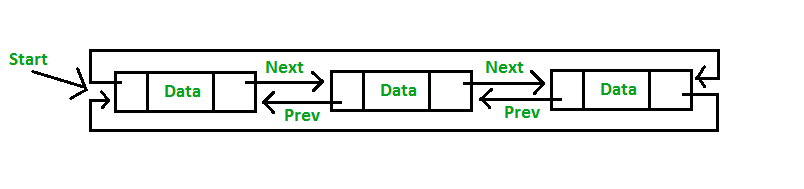Insertion in Circular Doubly Linked List

Insertion in Circular Doubly Linked List

• Insertion at the end of list or in an empty list
• Empty List (start = NULL): A node(Say N) is inserted with data = 5, so previous pointer of N points to N and next pointer of N also points to N. But now start pointer points to the first node the list.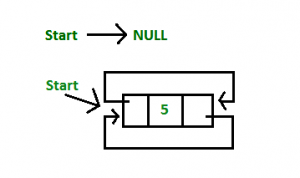• List initially contains some nodes, start points to first node of the List: A node(Say M) is inserted with data = 7, so previous pointer of M points to last node, next pointer of M points to first node and last node’s next pointer points to this M node and first node’s previous pointer points to this M node.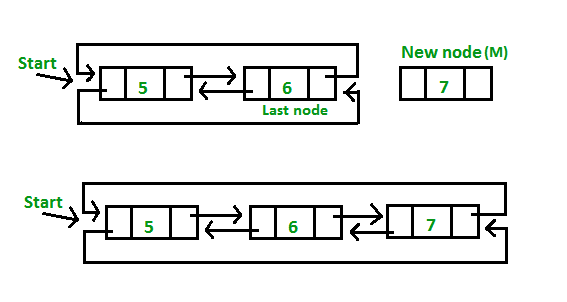C++

 // Function to insert at the endvoid insertEnd(struct Node** start, int value){    // If the list is empty, create a single node    // circular and doubly list    if (*start == NULL)    {        struct Node* new_node = new Node;        new_node->data = value;        new_node->next = new_node->prev = new_node;        *start = new_node;        return;    }     // If list is not empty     /* Find last node */    Node *last = (*start)->prev;     // Create Node dynamically    struct Node* new_node = new Node;    new_node->data = value;     // Start is going to be next of new_node    new_node->next = *start;     // Make new node previous of start    (*start)->prev = new_node;     // Make last previous of new node    new_node->prev = last;     // Make new node next of old last    last->next = new_node;}

Java

 // Function to insert at the endstatic void insertEnd(int value){         // If the list is empty, create a single      // node circular and doubly list    if (start == null)    {        Node new_node = new Node();        new_node.data = value;        new_node.next = new_node.prev = new_node;        start = new_node;        return;    }      // If list is not empty      // Find last node    Node last = (start).prev;      // Create Node dynamically    Node new_node = new Node();    new_node.data = value;      // Start is going to be      // next of new_node    new_node.next = start;      // Make new node previous of start    (start).prev = new_node;      // Make last previous of new node    new_node.prev = last;      // Make new node next of old last    last.next = new_node;} // This code is contributed by rutvik_56

Python3

 # Function to insert at the enddef insertEnd(value) :global start # If the list is empty, create a# single node circular and doubly listif (start == None) :     new_node = Node(0)    new_node.data = value    new_node.next = new_node.prev = new_node    start = new_node    return # If list is not empty # Find last node */last = (start).prev # Create Node dynamicallynew_node = Node(0)new_node.data = value # Start is going to be next of new_nodenew_node.next = start # Make new node previous of start(start).prev = new_node # Make last previous of new nodenew_node.prev = last # Make new node next of old lastlast.next = new_node # This code is contributed by shivanisinghss2110

C#

 // Function to insert at the endstatic void insertEnd(int value){    Node new_node;          // If the list is empty, create a single node    // circular and doubly list    if (start == null)    {        new_node = new Node();        new_node.data = value;        new_node.next = new_node.prev = new_node;        start = new_node;        return;    }      // If list is not empty      /* Find last node */    Node last = (start).prev;      // Create Node dynamically    new_node = new Node();    new_node.data = value;      // Start is going to be next of new_node    new_node.next = start;      // Make new node previous of start    (start).prev = new_node;      // Make last previous of new node    new_node.prev = last;      // Make new node next of old last    last.next = new_node;} // This code is contributed by Pratham76

Javascript


• Insertion at the beginning of the list: To insert a node at the beginning of the list, create a node(Say T) with data = 5, T next pointer points to first node of the list, T previous pointer points to last node the list, last node’s next pointer points to this T node, first node’s previous pointer also points this T node and at last don’t forget to shift ‘Start’ pointer to this T node.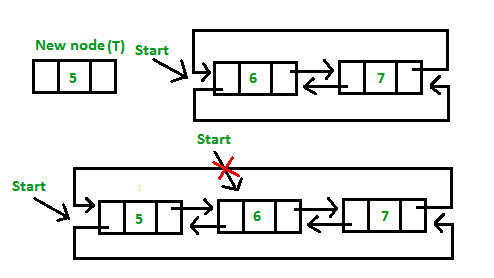C++

 // Function to insert Node at the beginning// of the List,void insertBegin(struct Node** start, int value){    // Pointer points to last Node    struct Node *last = (*start)->prev;     struct Node* new_node = new Node;    new_node->data = value;   // Inserting the data     // setting up previous and next of new node    new_node->next = *start;    new_node->prev = last;     // Update next and previous pointers of start    // and last.    last->next = (*start)->prev = new_node;     // Update start pointer    *start = new_node;}

Java

 // Function to insert Node at the beginning// of the List,static void insertBegin(int value){    // Pointer points to last Node    Node last = (start).prev;     Node new_node = new Node();    new_node.data = value; // Inserting the data     // setting up previous and next of new node    new_node.next = start;    new_node.prev = last;     // Update next and previous pointers of start    // and last.    last.next = (start).prev = new_node;     // Update start pointer    start = new_node;} // this code is contributed by shivanisinghss2110

Python3

 # Function to insert Node at the beginning# of the List,def insertBegin( value) :    global start         # Pointer points to last Node    last = (start).prev     new_node = Node(0)    new_node.data = value # Inserting the data     # setting up previous and    # next of new node    new_node.next = start    new_node.prev = last     # Update next and previous pointers    # of start and last.    last.next = (start).prev = new_node         # Update start pointer    start = new_node         # This code is contributed by shivanisinghss2110

C#

 // Function to insert Node at the beginning// of the List,static void insertBegin(int value){       // Pointer points to last Node    Node last = (start).prev;     Node new_node = new Node();    new_node.data = value; // Inserting the data     // setting up previous and next of new node    new_node.next = start;    new_node.prev = last;     // Update next and previous pointers of start    // and last.    last.next = (start).prev = new_node;     // Update start pointer    start = new_node;} // This code is contributed by shivanisinghss2110

Javascript

 // Function to insert Node at the beginning      // of the List,      function insertBegin(value) {        // Pointer points to last Node        var last = start.prev;         var new_node = new Node();        new_node.data = value; // Inserting the data         // setting up previous and next of new node        new_node.next = start;        new_node.prev = last;         // Update next and previous pointers of start        // and last.        last.next = start.prev = new_node;         // Update start pointer        start = new_node;      }             // This code is contributed by shivanisinghss2110
• Insertion in between the nodes of the list: To insert a node in between the list, two data values are required one after which new node will be inserted and another is the data of the new node.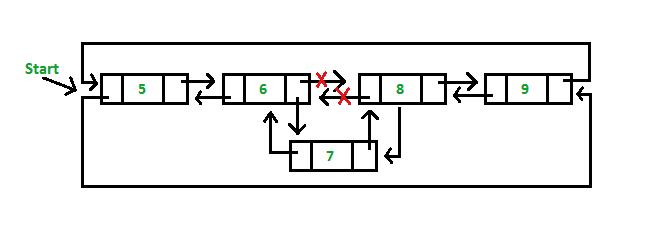C++

 // Function to insert node with value as value1.// The new node is inserted after the node with// with value2void insertAfter(struct Node** start, int value1,                                      int value2){    struct Node* new_node = new Node;    new_node->data = value1; // Inserting the data     // Find node having value2 and next node of it    struct Node *temp = *start;    while (temp->data != value2)        temp = temp->next;    struct Node *next = temp->next;     // insert new_node between temp and next.    temp->next = new_node;    new_node->prev = temp;    new_node->next = next;    next->prev = new_node;}

Java

 // Function to insert node with value as value1.// The new node is inserted after the node with// with value2static void insertAfter(int value1,                                    int value2){    Node new_node = new Node();    new_node.data = value1; // Inserting the data     // Find node having value2 and next node of it    Node temp = start;    while (temp.data != value2)        temp = temp.next;    Node next = temp.next;     // insert new_node between temp and next.    temp.next = new_node;    new_node.prev = temp;    new_node.next = next;    next.prev = new_node;} // this code is contributed by shivanisinghss2110

Python3

 # Function to insert node with value as value1.# The new node is inserted after the node with# with value2def insertAfter(value1, value2) :    global start    new_node = Node(0)    new_node.data = value1 # Inserting the data     # Find node having value2 and    # next node of it    temp = start    while (temp.data != value2) :        temp = temp.next    next = temp.next     # insert new_node between temp and next.    temp.next = new_node    new_node.prev = temp    new_node.next = next    next.prev = new_node     # this code is contributed by shivanisinghss2110

C#

 // Function to insert node with value as value1.// The new node is inserted after the node with// with value2static void insertAfter(int value1, int value2){    Node new_node = new Node();    new_node.data = value1; // Inserting the data     // Find node having value2 and next node of it    Node temp = start;    while (temp.data != value2)        temp = temp.next;    Node next = temp.next;     // insert new_node between temp and next.    temp.next = new_node;    new_node.prev = temp;    new_node.next = next;    next.prev = new_node;} // this code is contributed by shivanisinghss2110

Javascript



Following is a complete program that uses all of the above methods to create a circular doubly linked list.

C++

 // C++ program to illustrate inserting a Node in// a Circular Doubly Linked list in begging, end// and middle#include using namespace std; // Structure of a Nodestruct Node{    int data;    struct Node *next;    struct Node *prev;}; // Function to insert at the endvoid insertEnd(struct Node** start, int value){    // If the list is empty, create a single node    // circular and doubly list    if (*start == NULL)    {        struct Node* new_node = new Node;        new_node->data = value;        new_node->next = new_node->prev = new_node;        *start = new_node;        return;    }     // If list is not empty     /* Find last node */    Node *last = (*start)->prev;     // Create Node dynamically    struct Node* new_node = new Node;    new_node->data = value;     // Start is going to be next of new_node    new_node->next = *start;     // Make new node previous of start    (*start)->prev = new_node;     // Make last previous of new node    new_node->prev = last;     // Make new node next of old last    last->next = new_node;} // Function to insert Node at the beginning// of the List,void insertBegin(struct Node** start, int value){    // Pointer points to last Node    struct Node *last = (*start)->prev;     struct Node* new_node = new Node;    new_node->data = value;   // Inserting the data     // setting up previous and next of new node    new_node->next = *start;    new_node->prev = last;     // Update next and previous pointers of start    // and last.    last->next = (*start)->prev = new_node;     // Update start pointer    *start = new_node;} // Function to insert node with value as value1.// The new node is inserted after the node with// with value2void insertAfter(struct Node** start, int value1,                                      int value2){    struct Node* new_node = new Node;    new_node->data = value1; // Inserting the data     // Find node having value2 and next node of it    struct Node *temp = *start;    while (temp->data != value2)        temp = temp->next;    struct Node *next = temp->next;     // insert new_node between temp and next.    temp->next = new_node;    new_node->prev = temp;    new_node->next = next;    next->prev = new_node;}  void display(struct Node* start){    struct Node *temp = start;     printf("\nTraversal in forward direction \n");    while (temp->next != start)    {        printf("%d ", temp->data);        temp = temp->next;    }    printf("%d ", temp->data);     printf("\nTraversal in reverse direction \n");    Node *last = start->prev;    temp = last;    while (temp->prev != last)    {        printf("%d ", temp->data);        temp = temp->prev;    }    printf("%d ", temp->data);} /* Driver program to test above functions*/int main(){    /* Start with the empty list */    struct Node* start = NULL;     // Insert 5. So linked list becomes 5->NULL    insertEnd(&start, 5);     // Insert 4 at the beginning. So linked    // list becomes 4->5    insertBegin(&start, 4);     // Insert 7 at the end. So linked list    // becomes 4->5->7    insertEnd(&start, 7);     // Insert 8 at the end. So linked list    // becomes 4->5->7->8    insertEnd(&start, 8);     // Insert 6, after 5. So linked list    // becomes 4->5->6->7->8    insertAfter(&start, 6, 5);     printf("Created circular doubly linked list is: ");    display(start);     return 0;}

Java

 // Java program to illustrate inserting a Node in// a Circular Doubly Linked list in begging, end// and middleimport java.util.*; class GFG{ static Node start; // Structure of a Nodestatic class Node{    int data;    Node next;    Node prev;}; // Function to insert at the endstatic void insertEnd(int value){    // If the list is empty, create a single node    // circular and doubly list    if (start == null)    {        Node new_node = new Node();        new_node.data = value;        new_node.next = new_node.prev = new_node;        start = new_node;        return;    }     // If list is not empty     /* Find last node */    Node last = (start).prev;     // Create Node dynamically    Node new_node = new Node();    new_node.data = value;     // Start is going to be next of new_node    new_node.next = start;     // Make new node previous of start    (start).prev = new_node;     // Make last previous of new node    new_node.prev = last;     // Make new node next of old last    last.next = new_node;} // Function to insert Node at the beginning// of the List,static void insertBegin(int value){    // Pointer points to last Node    Node last = (start).prev;     Node new_node = new Node();    new_node.data = value; // Inserting the data     // setting up previous and next of new node    new_node.next = start;    new_node.prev = last;     // Update next and previous pointers of start    // and last.    last.next = (start).prev = new_node;     // Update start pointer    start = new_node;} // Function to insert node with value as value1.// The new node is inserted after the node with// with value2static void insertAfter(int value1,                                    int value2){    Node new_node = new Node();    new_node.data = value1; // Inserting the data     // Find node having value2 and next node of it    Node temp = start;    while (temp.data != value2)        temp = temp.next;    Node next = temp.next;     // insert new_node between temp and next.    temp.next = new_node;    new_node.prev = temp;    new_node.next = next;    next.prev = new_node;} static void display(){    Node temp = start;     System.out.printf("\nTraversal in forward direction \n");    while (temp.next != start)    {        System.out.printf("%d ", temp.data);        temp = temp.next;    }    System.out.printf("%d ", temp.data);     System.out.printf("\nTraversal in reverse direction \n");    Node last = start.prev;    temp = last;    while (temp.prev != last)    {        System.out.printf("%d ", temp.data);        temp = temp.prev;    }    System.out.printf("%d ", temp.data);} /* Driver code*/public static void main(String[] args){    /* Start with the empty list */    Node start = null;     // Insert 5. So linked list becomes 5.null    insertEnd(5);     // Insert 4 at the beginning. So linked    // list becomes 4.5    insertBegin(4);     // Insert 7 at the end. So linked list    // becomes 4.5.7    insertEnd(7);     // Insert 8 at the end. So linked list    // becomes 4.5.7.8    insertEnd(8);     // Insert 6, after 5. So linked list    // becomes 4.5.6.7.8    insertAfter(6, 5);     System.out.printf("Created circular doubly linked list is: ");    display();}} // This code is contributed by Rajput-Ji

Python3

 # Python3 program to illustrate inserting# a Node in a Circular Doubly Linked list# in begging, end and middlestart = None # Structure of a Nodeclass Node:    def __init__(self, data):        self.data = data        self.next = None        self.prev = None # Function to insert at the enddef insertEnd(value) :    global start         # If the list is empty, create a    # single node circular and doubly list    if (start == None) :         new_node = Node(0)        new_node.data = value        new_node.next = new_node.prev = new_node        start = new_node        return     # If list is not empty     # Find last node */    last = (start).prev     # Create Node dynamically    new_node = Node(0)    new_node.data = value     # Start is going to be next of new_node    new_node.next = start     # Make new node previous of start    (start).prev = new_node     # Make last previous of new node    new_node.prev = last     # Make new node next of old last    last.next = new_node # Function to insert Node at the beginning# of the List,def insertBegin( value) :    global start         # Pointer points to last Node    last = (start).prev     new_node = Node(0)    new_node.data = value # Inserting the data     # setting up previous and    # next of new node    new_node.next = start    new_node.prev = last     # Update next and previous pointers    # of start and last.    last.next = (start).prev = new_node     # Update start pointer    start = new_node # Function to insert node with value as value1.# The new node is inserted after the node with# with value2def insertAfter(value1, value2) :    global start    new_node = Node(0)    new_node.data = value1 # Inserting the data     # Find node having value2 and    # next node of it    temp = start    while (temp.data != value2) :        temp = temp.next    next = temp.next     # insert new_node between temp and next.    temp.next = new_node    new_node.prev = temp    new_node.next = next    next.prev = new_node def display() :    global start    temp = start     print ("Traversal in forward direction:")    while (temp.next != start) :         print (temp.data, end = " ")        temp = temp.next         print (temp.data)         print ("Traversal in reverse direction:")    last = start.prev    temp = last    while (temp.prev != last) :         print (temp.data, end = " ")        temp = temp.prev         print (temp.data) # Driver Codeif __name__ == '__main__':    global start         # Start with the empty list    start = None         # Insert 5. So linked list becomes 5.None    insertEnd(5)     # Insert 4 at the beginning. So linked    # list becomes 4.5    insertBegin(4)     # Insert 7 at the end. So linked list    # becomes 4.5.7    insertEnd(7)     # Insert 8 at the end. So linked list    # becomes 4.5.7.8    insertEnd(8)     # Insert 6, after 5. So linked list    # becomes 4.5.6.7.8    insertAfter(6, 5)     print ("Created circular doubly linked list is: ")    display() # This code is contributed by Arnab kundu

C#

 // C# program to illustrate inserting a Node in// a Circular Doubly Linked list in begging, end// and middleusing System;using System.Collections.Generic; class GFG{static Node start; // Structure of a Nodepublic class Node{    public int data;    public Node next;    public Node prev;}; // Function to insert at the endstatic void insertEnd(int value){    Node new_node;         // If the list is empty, create a single node    // circular and doubly list    if (start == null)    {        new_node = new Node();        new_node.data = value;        new_node.next = new_node.prev = new_node;        start = new_node;        return;    }     // If list is not empty     /* Find last node */    Node last = (start).prev;     // Create Node dynamically    new_node = new Node();    new_node.data = value;     // Start is going to be next of new_node    new_node.next = start;     // Make new node previous of start    (start).prev = new_node;     // Make last previous of new node    new_node.prev = last;     // Make new node next of old last    last.next = new_node;} // Function to insert Node at the beginning// of the List,static void insertBegin(int value){    // Pointer points to last Node    Node last = (start).prev;     Node new_node = new Node();    new_node.data = value; // Inserting the data     // setting up previous and next of new node    new_node.next = start;    new_node.prev = last;     // Update next and previous pointers of start    // and last.    last.next = (start).prev = new_node;     // Update start pointer    start = new_node;} // Function to insert node with value as value1.// The new node is inserted after the node with// with value2static void insertAfter(int value1, int value2){    Node new_node = new Node();    new_node.data = value1; // Inserting the data     // Find node having value2 and next node of it    Node temp = start;    while (temp.data != value2)        temp = temp.next;    Node next = temp.next;     // insert new_node between temp and next.    temp.next = new_node;    new_node.prev = temp;    new_node.next = next;    next.prev = new_node;} static void display(){    Node temp = start;     Console.Write("\nTraversal in forward direction \n");    while (temp.next != start)    {        Console.Write("{0} ", temp.data);        temp = temp.next;    }    Console.Write("{0} ", temp.data);     Console.Write("\nTraversal in reverse direction \n");    Node last = start.prev;    temp = last;    while (temp.prev != last)    {        Console.Write("{0} ", temp.data);        temp = temp.prev;    }    Console.Write("{0} ", temp.data);} /* Driver code*/public static void Main(String[] args){    /* Start with the empty list */    Node start = null;     // Insert 5. So linked list becomes 5.null    insertEnd(5);     // Insert 4 at the beginning. So linked    // list becomes 4.5    insertBegin(4);     // Insert 7 at the end. So linked list    // becomes 4.5.7    insertEnd(7);     // Insert 8 at the end. So linked list    // becomes 4.5.7.8    insertEnd(8);     // Insert 6, after 5. So linked list    // becomes 4.5.6.7.8    insertAfter(6, 5);     Console.Write("Created circular doubly linked list is: ");    display();}} // This code is contributed by Rajput-Ji

Javascript



Output:

Created circular doubly linked list is:
Traversal in forward direction
4 5 6 7 8
Traversal in reverse direction
8 7 6 5 4

• List can be traversed from both directions i.e. from head to tail or from tail to head.
• Jumping from head to tail or from tail to head is done in constant time O(1).
• Circular Doubly Linked Lists are used for the implementation of advanced data structures like Fibonacci Heap.

• It takes slightly extra memory in each node to accommodate the previous pointer.
• Lots of pointers involved while implementing or doing operations on a list. So, pointers should be handled carefully otherwise data of the list may get lost.

Applications of Circular doubly linked list

• Managing songs playlist in media player applications.
• Managing shopping cart in online shopping.

This article is contributed by Akash Gupta. If you like GeeksforGeeks and would like to contribute, you can also write an article using write.geeksforgeeks.org or mail your article to review-team@geeksforgeeks.org. See your article appearing on the GeeksforGeeks main page and help other Geeks.